The POWER Procedure
 TWOSAMPLEWILCOXON Statement

TWOSAMPLEWILCOXON <options> ;

The TWOSAMPLEWILCOXON statement performs power and sample size analyses for the Wilcoxon-Mann-Whitney test (also called the Wilcoxon rank-sum test, Mann-Whitney-Wilcoxon test, or Mann-Whitney U test) for two independent groups.

Note that the O’Brien-Castelloe approach to computing power for the Wilcoxon test is approximate, based on asymptotic behavior as the total sample size gets large. The quality of the power approximation degrades for small sample sizes; conversely, the quality of the sample size approximation degrades if the two distributions are far apart, so that only a small sample is needed to detect a significant difference. But this degradation is rarely a problem in practical situations, in which experiments are usually performed for relatively close distributions.

### Summary of Options

Table 68.25 summarizes categories of options available in the TWOSAMPLEWILCOXON statement.

Table 68.25 Summary of Options in the TWOSAMPLEWILCOXON Statement

Options

Define analysis

Specify analysis information

Specify distributions

Specify sample size and allocation

Specify power

Control sample size rounding

Specify computational options

Control ordering in output

Table 68.26 summarizes the valid result parameters in the TWOSAMPLEWILCOXON statement.

Table 68.26 Summary of Result Parameters in the TWOSAMPLEWILCOXON Statement

Analyses

Solve For

Syntax

TEST=WMW

Power

Sample size

### Dictionary of Options

ALPHA=number-list

specifies the level of significance of the statistical test. The default is 0.05, corresponding to the usual 0.05
100% = 5% level of significance. See the section Specifying Value Lists in Analysis Statements for information about specifying the number-list.

GROUPNS=grouped-number-list
GNS=grouped-number-list

specifies the two group sample sizes. See the section Specifying Value Lists in Analysis Statements for information about specifying the grouped-number-list.

GROUPWEIGHTS=grouped-number-list
GWEIGHTS=grouped-number-list

specifies the sample size allocation weights for the two groups. This option controls how the total sample size is divided between the two groups. Each pair of values for the two groups represents relative allocation weights. Additionally, if the NFRACTIONAL option is not used, the total sample size is restricted to be equal to a multiple of the sum of the two group weights (so that the resulting design has an integer sample size for each group while adhering exactly to the group allocation weights). Values must be integers unless the NFRACTIONAL option is used. The default value is (1 1), a balanced design with a weight of 1 for each group. See the section Specifying Value Lists in Analysis Statements for information about specifying the grouped-number-list.

NBINS=number-list

specifies the number of categories (or "bins") each variable’s distribution is divided into (unless it is ordinal, in which case the categories remain intact) in internal calculations. Higher values increase computational time and memory requirements but generally lead to more accurate results. However, if the value is too high, then numerical instability can occur. The default value is 1000. See the section Specifying Value Lists in Analysis Statements for information about specifying the number-list.

NFRACTIONAL
NFRAC

enables fractional input and output for sample sizes. See the section Sample Size Adjustment Options for information about the ramifications of the presence (and absence) of the NFRACTIONAL option.

NPERGROUP=number-list
NPERG=number-list

specifies the common sample size per group or requests a solution for the common sample size per group with a missing value (NPERGROUP=.). Use of this option implicitly specifies a balanced design. See the section Specifying Value Lists in Analysis Statements for information about specifying the number-list.

NTOTAL=number-list

specifies the sample size or requests a solution for the sample size with a missing value (NTOTAL=.). See the section Specifying Value Lists in Analysis Statements for information about specifying the number-list.

OUTPUTORDER=INTERNAL
OUTPUTORDER=REVERSE
OUTPUTORDER=SYNTAX

controls how the input and default analysis parameters are ordered in the output. OUTPUTORDER=INTERNAL (the default) arranges the parameters in the output according to the following order of their corresponding options:

The OUTPUTORDER=SYNTAX option arranges the parameters in the output in the same order in which their corresponding options are specified in the TWOSAMPLEWILCOXON statement. The OUTPUTORDER=REVERSE option arranges the parameters in the output in the reverse of the order in which their corresponding options are specified in the TWOSAMPLEWILCOXON statement.

POWER=number-list

specifies the desired power of the test or requests a solution for the power with a missing value (POWER=.). The power is expressed as a probability, a number between 0 and 1, rather than as a percentage. See the section Specifying Value Lists in Analysis Statements for information about specifying the number-list.

SIDES=keyword-list

specifies the number of sides (or tails) and the direction of the statistical test. Valid keywords are as follows:

1

one-sided with alternative hypothesis in same direction as effect

2

two-sided

U

upper one-sided with alternative greater than null value

L

lower one-sided with alternative less than null value

The default value is 2.

TEST=WMW

specifies the Wilcoxon-Mann-Whitney test for two independent groups This is the default test option.

VARDIST("label")=distribution (parameters)

defines a distribution for a variable.

For the VARDIST= option,

label

identifies the variable distribution in the output and with the VARIABLES= option.

distribution

specifies the distributional form of the variable.

parameters

specifies one or more parameters associated with the distribution.

Choices for distributional forms and their parameters are as follows:

ORDINAL ((values) : (probabilities))

is an ordered categorical distribution. The values are any numbers separated by spaces. The probabilities are numbers between 0 and 1 (inclusive) separated by spaces. Their sum must be exactly 1. The number of probabilities must match the number of values.

BETA (a, b <, l, r >)

is a beta distribution with shape parametersandand optional location parametersand. The values ofandmust be greater than 0, andmust be less than. The default values forandare 0 and 1, respectively.

BINOMIAL (p, n)

is a binomial distribution with probability of successand number of independent Bernoulli trials. The value ofmust be greater than 0 and less than 1, andmust be an integer greater than 0.

EXPONENTIAL (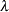)

is an exponential distribution with scale, which must be greater than 0.

GAMMA (a,)

is a gamma distribution with shapeand scale. The values ofandmust be greater than 0.

LAPLACE (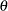,)

is a Laplace distribution with locationand scale. The value ofmust be greater than 0.

LOGISTIC (,)

is a logistic distribution with locationand scale. The value ofmust be greater than 0.

LOGNORMAL (,)

is a lognormal distribution with locationand scale. The value ofmust be greater than 0.

NORMAL (,)

is a normal distribution with meanand standard deviation. The value ofmust be greater than 0.

POISSON (m)

is a Poisson distribution with mean. The value ofmust be greater than 0.

UNIFORM (l, r)

is a uniform distribution on the interval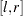, where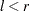.

VARIABLES=grouped-name-list
VARS=grouped-name-list

specifies the distributions of two or more variables, using labels specified with the VARDIST= option. See the section Specifying Value Lists in Analysis Statements for information about specifying the grouped-name-list.

### Restrictions on Option Combinations

To specify the sample size and allocation, choose one of the following parameterizations:

• sample size per group in a balanced design (using the NPERGROUP= option)

• total sample size and allocation weights (using the NTOTAL= and GROUPWEIGHTS= options)

• individual group sample sizes (using the GROUPNS= option)

### Option Groups for Common Analyses

This section summarizes the syntax for the common analyses supported in the TWOSAMPLEWILCOXON statement.

#### Wilcoxon-Mann-Whitney Test for Comparing Two Distributions

The following statements performs a power analysis for Wilcoxon-Mann-Whitney tests comparing an ordinal variable with each other type of distribution. Default values for the ALPHA=, NBINS=, SIDES=, and TEST= options specify a two-sided test with a significance level of 0.05 and the use of 1000 categories per distribution when discretization is needed.

```proc power;
twosamplewilcoxon
vardist("myordinal") = ordinal ((0 1 2) : (.2 .3 .5))
vardist("mybeta1") = beta (1, 2)
vardist("mybeta2") = beta (1, 2, 0, 2)
vardist("mybinomial") = binomial (.3, 3)
vardist("myexponential") = exponential (2)
vardist("mygamma") = gamma (1.5, 2)
vardist("mylaplace") = laplace (1, 2)
vardist("mylogistic") = logistic (1, 2)
vardist("mylognormal") = lognormal (1, 2)
vardist("mynormal") = normal (3, 2)
vardist("mypoisson") = poisson (2)
vardist("myuniform") = uniform (0, 2)
variables = "myordinal" | "mybeta1" "mybeta2" "mybinomial"
"myexponential" "mygamma" "mylaplace"
"mylogistic" "mylognormal" "mynormal"
"mypoisson" "myuniform"
ntotal = 40
power = .;
run;
```Previous Page | Next Page | Top of Page